• 解决任务的思路是借助“查找和替换”来间接实现条件格式功能，操作步骤如下：第1步：调出“查找和替换”对话框这里要说明的是，我们从“格式”菜单下是找不到设置条件格式相关选项的，word毕竟与Excel有所不同。...解决任务的思路是借助“查找和替换”来间接实现条件格式功能，操作步骤如下：

第1步：调出“查找和替换”对话框这里要说明的是，我们从“格式”菜单下是找不到设置条件格式相关选项的，word毕竟与Excel有所不同。正确的步骤是从“编辑”菜单的格式菜单下选择“查找”或“替换”命令，这两条命令都可以打开“查找和替换”对话框，前者是默认显示“查找”标签页，后者是默认显示“替换”标签页，操作更方便一些。

第2步：设置条件如果整篇文档中只有很少的几个英文单词，那么设置起来并不复杂，最多手工设置后通过“格式刷”的功能复制一下就可以了。但现在我们的目的是为文档中的所有英文内容设置特定的格式，而且文档中的英文内容非常繁复，手工操作显然并不现实。

仍旧按照常规的方法进行操作，首先将光标定位在“查找内容”框，然后在“特殊字符”下拉菜单中选择“任意字母”，也可以手工输入“^”。接下来，将光标定位在“替换为”框，然后在“格式”下拉菜单中选择“字体”或其他选项进行设置，例如笔者在这里将所有英文内容的格式设置为Arial Unicode MS字体、大小11磅、不加粗、不倾斜、字体颜色为蓝色，目的是为了便于与中文内容区别开来。 第3步：确认替换完成上述设置后，单击“全部替换”按钮即可将文档中的所有英文内容设置为特定的格式，现在看起来就清晰多了。当然，如果你不满意，可以重新打开“查找和替换”对话框进行设置，这里就不再多介绍，感兴趣的朋友不妨自行试验。 小提示：使用通配符实现“条件格式” 上述功能我们还可以使用通配符来实现，在“查找内容”框中输入“[a-z，A-Z]”，这行代码的含义是查找所有英文字符，“a-z”表示所有小写的英文字符，“A-Z”表示所有大写的英文字符，“替换为”仍旧按照前面的格式进行设置，但关键是要将搜索选项中的“使用通配符”复选框选中，最后单击“全部替换”按钮即可完成。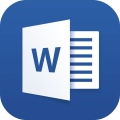Word中如何实现“条件格式”.doc 下载Word文档到电脑，方便收藏和打印[全文共795字] 编辑推荐：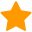下载Word文档 展开全文• 本篇是使用openpyxl库的一点经验总结，涉及到以下功能：操作excel、设置边框背景颜色居中等格式、设置数字格式（小数百分数）、百分比条件格式、插入行列等，依然是代码+注释+总结。 任务目标：批量调整excel格式，... #简介# 本篇是使用openpyxl库的一点经验总结，涉及到以下功能：操作excel、设置边框背景颜色居中等格式、设置数字格式（小数百分数）、百分比条件格式、插入行列等，依然是代码+注释+总结 任务目标：批量调整excel格式，并设置条件格式 #代码展示# #仅展示思路，代码做了简化 import pandas as pd import openpyxl from openpyxl import Workbook,load_workbook from openpyxl.utils.dataframe import dataframe_to_rows from openpyxl.styles import PatternFill,Font,Color,PatternFill,Alignment,numbers,Border,Side from openpyxl.formatting.rule import ColorScaleRule, CellIsRule, FormulaRule import os,sys import numpy as np def walk(path): if not os.path.exists(path): return -1 for root,dirs,names in os.walk(path): for filename in names: if os.path.splitext(filename) == '.xlsx': doc = os.path.join(root,filename) print(doc) csvlist.append(doc) #确定当前所在的文件夹路径 csvlist = [] cur_path = os.getcwd() walk(cur_path) def my_border(t_border, b_border, l_border, r_border): border = Border(top=Side(border_style=t_border, color="000000"), bottom=Side(border_style=b_border, color="000000"), left=Side(border_style=l_border, color="000000"), right=Side(border_style=r_border, color="000000")) return border def format_border(s_column, s_index, e_column , e_index): for row in tuple(sheet[s_column + str(s_index):e_column + str(e_index)]): for cell in row: cell.border = my_border('thin', 'thin', 'thin', 'thin') redFill = PatternFill(start_color='FFC0CB',end_color='FFC0CB',fill_type='solid') rule_1=ColorScaleRule(start_type='percentile',start_value=0,start_color='FF8000',mid_type='percentile',mid_value=20,mid_color='FF8000',end_type='percentile',end_value=21,end_color='FFFFFF') rule_2=ColorScaleRule(start_type='percentile',start_value=79,start_color='FFFFFF',mid_type='percentile',mid_value=80,mid_color='FF8000',end_type='percentile',end_value=100,end_color='FF8000') for doc in csvlist: wb = openpyxl.load_workbook(doc) ws = wb.active for cell in ws["F"]: cell.number_format = "0.00%" cell.alignment = Alignment(horizontal='center', vertical='center') for cell in ws["G"]: cell.number_format = "0.00%" cell.alignment = Alignment(horizontal='center', vertical='center') for cell in ws["I"]: cell.number_format = '0' cell.alignment = Alignment(horizontal='center', vertical='center') for cell in ws["1"]: cell.alignment = Alignment(wrapText=True,horizontal='center', vertical='center') cell.fill = PatternFill("solid",fgColor="009900") cell.font = Font(bold=True,color ="FFFFFF",size=11) ws.column_dimensions['A'].width = 10 ws.conditional_formatting.add('D2:D99',rule_1) ws.conditional_formatting.add('E2:E99',rule_2) sheet = ws row=ws.max_row column=ws.max_column format_border('A', 2,"S",row) ws.conditional_formatting.add('E2:E34',rule_1) ws.insert_rows(1) ws.merge_cells('A1:S1') ws["A1"]='=A3&"战队指标展板公示"&TEXT(EOMONTH(TODAY(),-1)+1,"yyyy/mm/dd")&"-"&TEXT(TODAY()-1,"yyyy/mm/dd")&""' ws["A1"].font = Font(bold=True,color ="FFFFFF",size=16) ws["A1"].alignment = Alignment(horizontal='center', vertical='center') fill_1 = PatternFill("solid",fgColor="009900") ws["A1"].fill = fill_1 doc1 = os.path.splitext(doc) wb.save(doc1+"-修改.xlsx") wb.close() #代码拆解# 遍历文件夹、读取excel部分与前篇相同，此处不做赘述。 打开保存关闭excel #打开excel，获取excel里的表，选中某一个sheet进行操作 wb = openpyxl.load_workbook(doc) print wb.sheetnames ws = wb["Sheet1"] ws = wb.active #创建工作表（0是第一张表），设置表名和颜色 wb = Workbook() ws = wb.create_sheet("Mysheet", 0) ws.title = "New Title" ws.sheet_properties.tabColor = "1072BA" #保存和关闭excel,此时默认直接覆盖同名excel wb.save('test.xlsx') wb.close() #保存多页sheet的写入方法 with pd.ExcelWriter(输出文件名) as writer: df_order.to_excel(writer,sheet_name='订单表',index=False) data_total_t.to_excel(writer,sheet_name='战队指标',index=False) #与pandas联动,从dataframe里读取表 from openpyxl.utils.dataframe import dataframe_to_rows wb = Workbook() ws = wb.active for r in dataframe_to_rows(df, index=True, header=True): ws.append(r) 插入合并单元格，设置单元格样式 #在第一行插入行，合并A1到S1 ws.insert_rows(1) ws.merge_cells('A1:S1') #输入excel公式，效果是“XX战队指标展板公示（本月月初-昨日）” #eomonth（today(),-1）输出本月月初日，TEXT()公式设置时间日期格式 #将公式与excel里的引号用不同的引号区分，方便py识别 ws["A1"]='=A3&"战队指标展板公示"&TEXT(EOMONTH(TODAY(),-1)+1,"yyyy/mm/dd")&"-"&TEXT(TODAY()-1,"yyyy/mm/dd")&""' #设置文字格式、居中、背景填充,font是文字格式,alignment是对齐,patternfill是填充 ws["A1"].font = Font(name=u'微软雅黑',bold=True,color ="FFFFFF",size=16) ws["A1"].alignment = Alignment(horizontal='center', vertical='center') fill_1 = PatternFill("solid",fgColor="009900") ws["A1"].fill = fill_1 #设置行高列宽,列宽大约一个字号11的汉字占用2 ws.column_dimensions['A'].width = 10 ws.row_dimensions.height = 40 #设置整列的数字类型,"0.00%"是百分数,保留两位小数 #时间日期类型待补充，对多列进行操作暂未找出便捷方法 for cell in ws["I"]: cell.number_format = '0' cell.alignment = Alignment(horizontal='center', vertical='center') #pandas里的改变数字类型： 保留两位小数 df.round(2) 设置百分数 df['comment'].map(lambda x:format(x,'.2%')) 千位分隔符 df['view'].map(lambda x:format(x,',')) 设置边框 #定义边框类型和颜色 def my_border(t_border, b_border, l_border, r_border): border = Border(top=Side(border_style=t_border, color="000000"), bottom=Side(border_style=b_border, color="000000"), left=Side(border_style=l_border, color="000000"), right=Side(border_style=r_border, color="000000")) return border #定义加边框的单元格范围 def format_border(s_column, s_index, e_column , e_index): for row in tuple(sheet[s_column + str(s_index):e_column + str(e_index)]): for cell in row: cell.border = my_border('thin', 'thin', 'thin', 'thin') #对从A2到对角加边框 sheet = ws row=ws.max_row column=ws.max_column format_border('A', 2,column,row) 设置条件格式 #使用的openpyxl自带三色阶功能实现的条件格式，以百分比形式进行色阶填充，两端色阶是对从最小值到最大值进行填充，三色阶是对值1-值2进行一次过渡填充，值2-值3做一次过渡填充 #为了仿效excel里标记后20%的条件格式，设置了从0%开始到20%用同样的颜色，第三个值选择21%，填充白色，只要数据量不过分大，是不会出现20-21之间的过渡色的 rule_1=ColorScaleRule(start_type='percentile',start_value=0,start_color='FF8000',mid_type='percentile',mid_value=20,mid_color='FF8000',end_type='percentile',end_value=21,end_color='FFFFFF') rule_2=ColorScaleRule(start_type='percentile',start_value=79,start_color='FFFFFF',mid_type='percentile',mid_value=80,mid_color='FF8000',end_type='percentile',end_value=100,end_color='FF8000') ws.conditional_formatting.add('E2:E34',rule_1) 以上就是本次分享，欢迎关注，不定期更新 展开全文python excel 数据分析 • Excel 条件格式实现甘特图工作日条件格式设置添加条件格式周未条件格式设置 为了讲解公式截个效果图参考。日期排的不合理请无视。 工作日效果：有工作【紫色██】，无工作【白色██】 周未日效果：有工作【紫色█... Excel 条件格式实现甘特图 为了讲解公式截个效果图参考。日期排的不合理请无视。 工作日效果：有工作【紫色██】，无工作【白色██ 周未日效果：有工作【紫色██】，无工作【灰色██】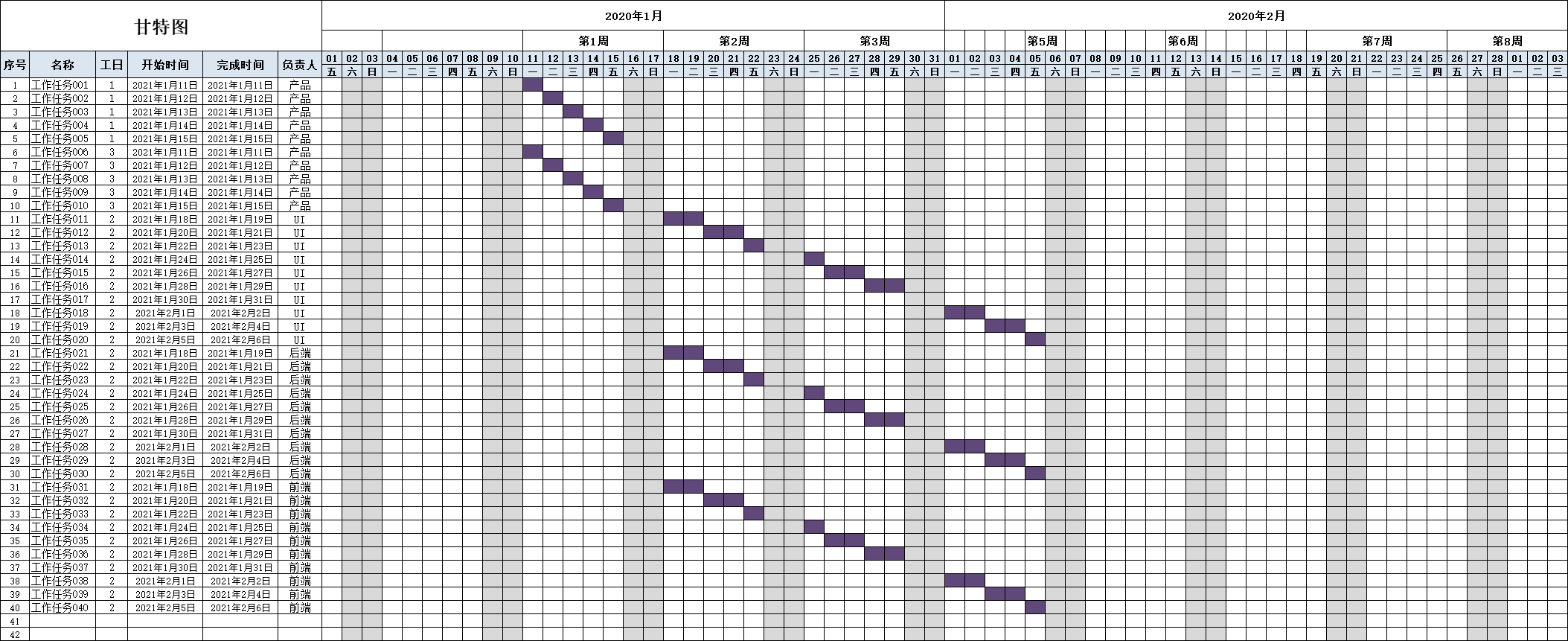工作日条件格式设置 添加条件格式 框选要设置的区域 =G$5:$BP$46》开始》条件格式》新建规则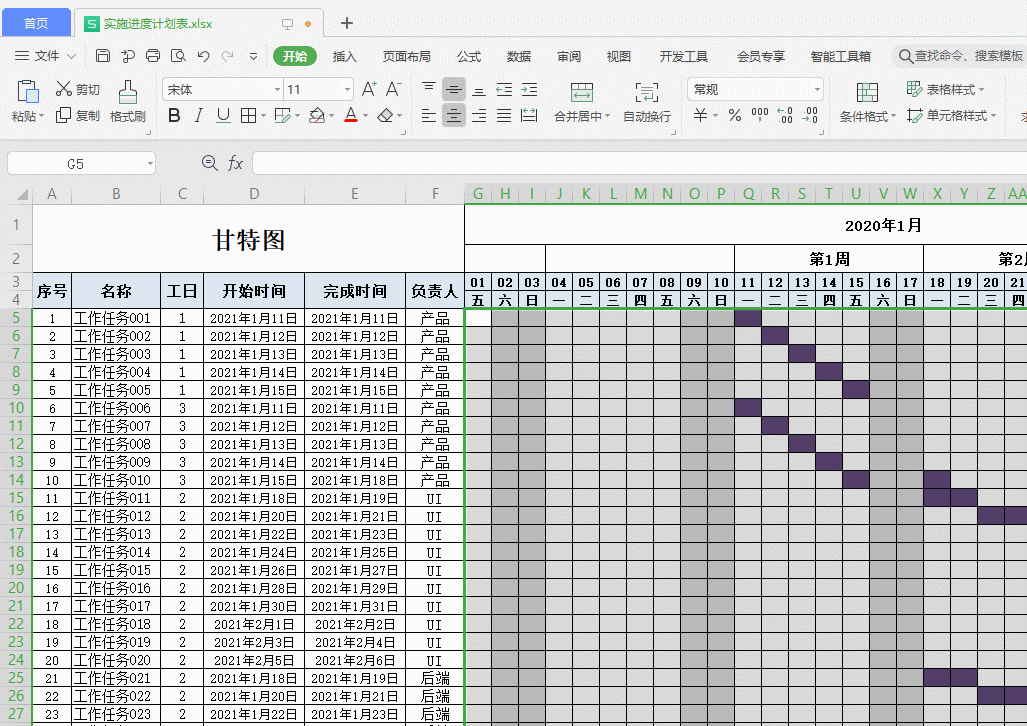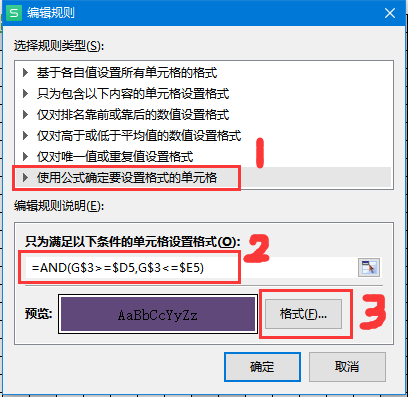1. 选择条件格式规则 2. 添加工作日条件公式=AND(G$3>=$D5,G$3<=$E5) 当前单元格的值满足： >=$D5 并且 <=$E5 条件生效。 3. 设置单元格满足条件生效的格式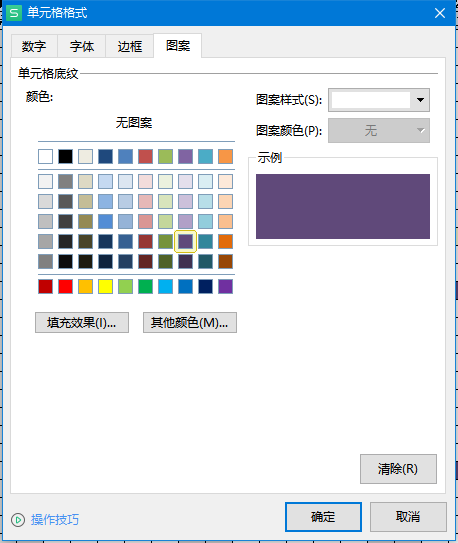条件优先级 列表中从上到下：从高到低。（两个小三角按钮可以调整顺序）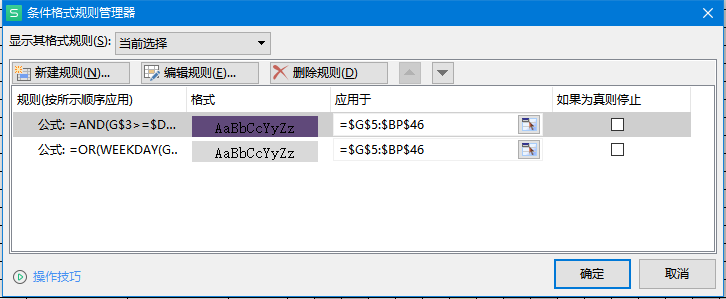周未条件格式设置 周未条件公式：=OR(WEEKDAY(G$4,2)=6,WEEKDAY(G\$4,2)=7)
操作步骤参考前面。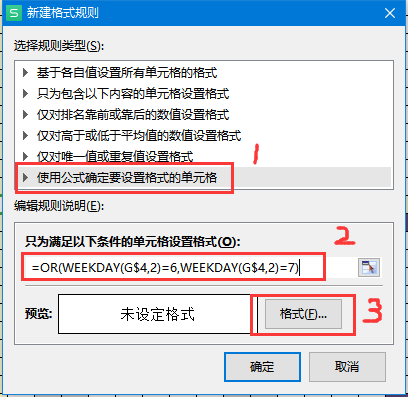展开全文excel 条件格式 公式
• 我可以使用条件格式设置单元格背景颜色，但我希望根据一个单元格文本值设置整行背景。在例如：import pandas as pdimport numpy as npimport xlsxwriterimport osdef main():xlsfilename = os.path.expanduser("~") ...

我可以使用条件格式设置单元格背景颜色，但我希望根据一个单元格文本值设置整行背景。在

例如：import pandas as pd

import numpy as np

import xlsxwriter

import os

def main():

xlsfilename = os.path.expanduser("~") + "/test.xls"

writer = pd.ExcelWriter(xlsfilename,engine='xlsxwriter')

create_sheet(writer)

set_format(writer)

writer.save()

return

def create_sheet(writer):

index = np.arange(1)

df = pd.DataFrame(columns=["Col1","Col2","Col3"],index=index)

index = 0

df.ix[index] = ["completed","(1,2)","(1,3)"]

index += 1

df.ix[index] = ["pending","(2,2)","(2,3)"]

index += 1

index += 1

df.to_excel(writer,sheet_name="MySheet",index=False)

return

def set_format(writer):

# Get the xlsxwriter objects from the dataframe writer object.

workbook = writer.book

worksheets = writer.sheets

'bold': False,

'border': 6,

'align': 'center',

'valign': 'vcenter',

'bg_color': '#D7E4BC',

})

for item in worksheets:

print item

worksheet = worksheets[item]

worksheet.set_column('A:A',10)

worksheet.set_column('B:B',10)

worksheet.set_column('C:C',10)

worksheet.data_validation('A1', {'validate': 'list',

worksheet.conditional_format('A1:A1000', {'type': 'text',

'criteria': 'begins with',

'value': 'completed',

'format': completed_fmt})

workbook.close()

return

#-----------------------------------------#

if __name__ == "__main__":

main()

#-----------------------------------------#

当前代码仅在单元格为文本“completed”时设置背景。但我希望如果单元格A文本是“完成的”，那么设置A，B，C背景颜色相同的值。在

电流输出为：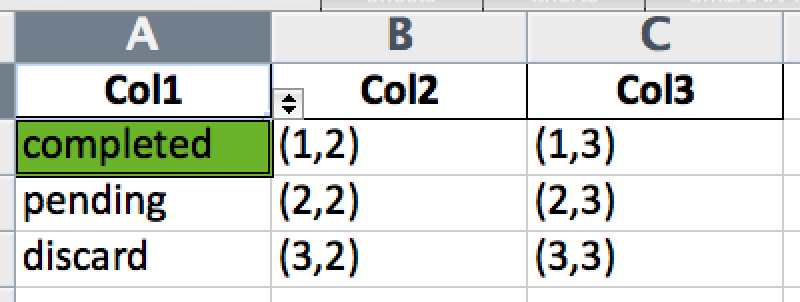展开全文• 一、使用简单的条件格式 1、为特定范围的数值标记特殊颜色 2、查找重复值 3、为数据透视表中的数据制作数据条 二、定义多重条件的条件格式 1、如何管理条件 2、创建多重条件 3、多重条件的优先级问题 三、...excel
• VBA条件格式的原理 利用VBA实现条件格式设置的过程并不像在Excel工作表中设置单元格条件格式那样方便。在VBA中，对于批量操作，通常需要用循环结构逐个进行。 此处，初学者一般能够掌握为满足条件的数据设置颜色...
• 文章目录前言一、条件格式 前言 筛选功能能够筛选出符合条件的数据，但是不符合条件的数据不予显示。条件格式能够将符合条件的数据以颜色、字体等方式标注出来，让我们能够更容易地注意到这些数据。 一、条件格式 ...excel 数据分析
• 今天同事提了一个需求，结果如下图所示。 备注里面为空或者为“✔”则为白底，出现任意其他字符就使用红色填充。 操作步骤 公式分析一下： ISBLANK()判断单元格是不是空的（如果是#N/A就需要ISNA()来判断） ...excel
• 条件格式 | 新建格式 | 只为包含以下内容的单元格设置格式 | 单元格值 介于 =TODAY()-2 =TODAY() 规则二(超过截止日期)： 条件格式 | 新建格式 | 只为包含以下内容的单元格设置格式 | 单元格值 大于 =TODAY() ...
• Excel基础(14)条件格式与公式 一.条件格式 1.1.某数据大于一个数 1.2.重看重复值 1.3.文本包含 1.4.多情况标记颜色？ 多次添加条件格式即可 1.5.金额大于数值标记日期突出显示？ 1.6.错误值不显示 1.7....机器学习 mysql python
• 获取想要设置格式的数据 import pandas as pd df=pd.read_csv("C:\\Users\\Desktop\\xz.csv",encoding='GBK',header=None) df1=df.loc[:,1:5] df1.columns=['xc','xr','xx','cx','sq'] import numpy as np df1.loc[4...python 运维
• 当我们希望数据看起来更醒目，会给数据加上特殊的标记，比如小红旗或星星之类的。这个操作在Excel中，是很方便的，只要使用条件格式中的图标...Excel用条件格式给数据插上小红旗默认规则在条件格式的最下面选管理规...
•excel
• 【 ee21.cn - excel 】用Excel条件格式给成绩不及格同学亮黄牌教师们通常会利用Excel电子表格对学生的成绩做系统的对比分析。为了查看方便，我们可以利用...)单击菜单栏格式→条件格式(如图1)，打开条件格式对话框...
• 原标题：计算机二级Excel条件格式的8大用法，太全了！Excel中条件格式这个功能，大家都不陌生，它的功能也非常强大，今天我们来个大盘点：1、显示重复值选中数据区域——开始——条件格式——突出显示单元格规则——...
• 》仅仅只是介绍了单元格图形格式中的“数据条”。有了那个基础之后，再来学习“色阶”和“图标集”就更简单了。“色阶”的用法和“数据条”完全相同，只不过它更常用于表现“热图”性质的数据，比如气温的变化、销售...
• wps js宏操作【条件格式】

千次阅读 2021-06-26 01:07:20wps javascript
• mysql查询记录如果有时间戳字段时，查看结果不方便，不能即时看到时间戳代表的含义，现提供mysql格式换时间函数，可以方便的看到格式化后的时间。1. DATE_FORMAT() 函数用于以不同的格式显示日期/时间数据。DATE_...
• 对于刚刚接触到python这门编程语言的朋友来说，刚刚开始学习python时候，对于python中的条件语句的了解不多，在这篇文章之中我们就来讲解一下关于python条件语句和条件语句的一般格式这方面的知识吧。python条件语句...
• matlabif条件语句

千次阅读 2021-04-24 13:05:24
matlab if语句在MATLAB中，if语句有3种格式。(1) 单分支if语句：if 条件 语句组 end当条件成立时，则执行语句组，执行完之后继续执行if语句的后继语句，若条件不成立，则直接执行if语句的后继语句。 (2) 双分支if...
• oracle查询日期格式转化

千次阅读 2021-03-12 19:33:15
# to_char:将日期格式化为指定格式,to_number:将字符串格式化为数值类型,YYYYMMDDHH24MISS:可以转化为其他格式的,注意是12小时制的还是24小时制的,依据实际情况决定,比如where条件后的是24小时制的，在这里就是24...
• QueryWrapper日期格式化mysql

千次阅读 2021-09-03 16:38:42
// Mysql String dateStr = "2021-09-03 16:37:52" QueryWrapper<UserInfo> wrapper = new QueryWrapper<>(); wrapper.eq("REPAY_STS_CD", "1"); wrapper.apply("DATE(CRT_TM) <...java
• 首先举个栗子（不想看的话直接下面看总结）： ...sum函数中使用if判断条件：{ sum( IF (order_type = 0, real_price, 0) ) AS ‘当天支付收入’, sum( IF (order_type = 1, real_price, 0) ) AS ‘当天打赏收入’, }mysql
• 前言开发过程中遇到了一个需求，根据用户选择的联系方式，动态改变输入框的检验条件，并且整个表单是可以增加的思考几个问题1.整个表单是可新增的，所以要遍历生成；2.联系方式(手机/座机)的切换，是要切换后边不同...
• 前言开发过程中遇到了一个需求，根据用户选择的联系方式，动态改变输入框的检验条件，并且整个表单是可以增加的思考几个问题1.整个表单是可新增的，所以要遍历生成；2.联系方式(手机/座机)的切换，是要切换后边不同...
• Py之pandas：利用where、replace等函数对dataframe格式数据按照条件进行数据替换 目录 利用where、replace等函数对dataframe格式数据按照条件进行数据替换 利用where、replace等函数对dataframe格式数据...
• 如下操作： 条件格式 选中将要处理的数据区域，点击菜单栏里【开始】——【条件格式】里选择【突出显示单元格规则】——【重复值】。如下操作： 数据透视表 数据透视表一样能计算数据重复的频次。而且比COUNTIF更加...Python 数据分析 Excel
• 条件运算符也叫做三元运算符，语法格式条件表达式 ? 语句1 : 语句2 true ? console.log('我是语句1') : console.log('我是语句2'); // 输出结果为我是语句1 false ? console.log('我是语句1') : console.log('我...javascript
• ** Vue+element UI的动态表单的校验(根据条件动态切换校验格式)** 1.整个表单是可新增的，所以要遍历生成； 2.因为input 是动态生成的，所以检验规则也需要动态生成 实现 elementui的form表单实现校验的时候要给当前...vue javascript js vue.js...